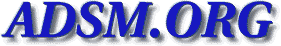Veritas-bu [Top] [All Lists]

## [Veritas-bu] Complete Veritas US Price List

2005-08-04 11:34:37
 Subject: [Veritas-bu] Complete Veritas US Price List Tammy.Yosifovski AT usfc DOT com (Yosifovski, Tammy) Thu, 4 Aug 2005 11:34:37 -0400
 ```This is a multi-part message in MIME format. ------_=_NextPart_001_01C5990A.060A4990 Content-Type: text/plain; charset="US-ASCII" Content-Transfer-Encoding: quoted-printable Anyone have a complete/current Veritas US Price list of all of their=0D=0Ap= roductions that they can pass along=3F I need MSRPs for Netbackup 5.X.=20=0D= =0A=0D=0AThank you!=0D=0A=0D=0A=0D=0A=0D=0A=0D=0A--------------------------= -----------------------------------------------------------=0D=0AThis e-mai= l is the exclusive, private and confidential property of the sender. The=0D= =0Ainformation contained in it is intended solely for the use of the sender= and the=0D=0Aintended recipient. If you are not the intended recipient you= are hereby advised that=0D=0Aany unauthorized disclosure, copying, distrib= ution or the taking of any action in=0D=0Areliance on the contents of this = message is strictly prohibited. If you have received=0D=0Athis e-mail in er= ror, please notify us immediately by telephone (call the USF=0D=0ACorporati= on Technical Support Center at 1-888-873-8721) and then destroy this=0D=0Ad= ocument and any copies in any form immediately. Finally, the recipient sho= uld=0D=0Acheck this email and any attachments for the presence of viruses. = USF Corporation=0D=0Aaccepts no liability for any damage caused by any viru= s transmitted by this email.=0D=0A-----------------------------------------= --------------------------------------------=0D=0A=0D=0A ------_=_NextPart_001_01C5990A.060A4990 Content-Type: text/html; charset="US-ASCII" Content-Transfer-Encoding: quoted-printable =0D=0A=0D=0A=0D= =0A=0D=0A=0D=0AComplete Veritas US Price List=0D=0A=0D= =0A=0D=0A=0D=0A=0D=0A

Anyone have a complete/current Veritas US Price lis= t of all of their productions that they can pass along=3F  I need MSRP= s for Netbackup 5.X.

=0D=0A=0D=0A

Thank you!=0D=0A

=0D=0A
=0D=0A
=0D=0A
=0D=0A=0D=0A
=

--------------= -----------------------------------------------------------------------
=0D= =0AThis e-mail is the exclusive, private and&= nbsp;confidential property of the sender. The
=0D= =0Ainformation contained in it is intended so= lely for the use of the sender and = the
=0D=0Aintended recipient. If you are not&nb= sp;the intended recipient you are hereby advi= sed that
=0D=0Aany unauthorized disclosure, copying,=  distribution or the taking of any actio= n in
=0D=0Areliance on the contents of thi= s message is strictly prohibited. If you = ;have received
=0D=0Athis e-mail in error, plea= se notify us immediately by telephone (call&n= bsp;the USF
=0D=0ACorporation Technical Support Cent= er at 1-888-873-8721) and then destroy this=0D=0Adocument and any copies in any form&n= bsp;immediately.  Finally, the recipient should=0D=0Acheck this email and any attachments f= or the presence of viruses. USF Corporation=0D=0Aaccepts no liability for any damage c= aused by any virus transmitted by this e= mail.
=0D=0A------------------------------------------------------------= -------------------------
=0D=0A
=0D=0A
=0D=0A= ------_=_NextPart_001_01C5990A.060A4990-- ```
 Current Thread [Veritas-bu] Complete Veritas US Price List, Yosifovski, Tammy <= [Veritas-bu] Complete Veritas US Price List, Shawn_Coleman AT booksarefun DOT com [Veritas-bu] Complete Veritas US Price List, Piszcz, Justin